## IntroductionThis vignette shows some examples how to set up different inference models in `babette`.

For all examples, do load `babette`:

``library(babette)``

All these examples check that `BEAST2` is installed at the default location at /github/home/.local/share/beast/lib/launcher.jar. If this is not the case, we will use some fabricated output:

``````posterior <- create_test_bbt_run_output()
posterior\$anthus_aco_sub_trees <- posterior\$anthus_aco_trees
names(posterior)
#>  "estimates"            "anthus_aco_trees"     "operators"
#>  "output"               "anthus_aco_sub_trees"``````

All examples read the alignment from a FASTA file (usually `my_fasta.fas`).

``fasta_filename <- get_babette_path("anthus_aco_sub.fas")``

Instead of a full run, the MCMC chain length is shortened to 10K states, with a measurement every 1K states:

``mcmc <- create_test_mcmc(chain_length = 10000)``

We will re-create this MCMC setup, as doing so initializes it with new filenames for temporary files. These temporary files should not exist before a run and should exist after a run. Sure, there is the option to overwrite…

## Example #1: all default

Using all default settings, only specify a DNA alignment.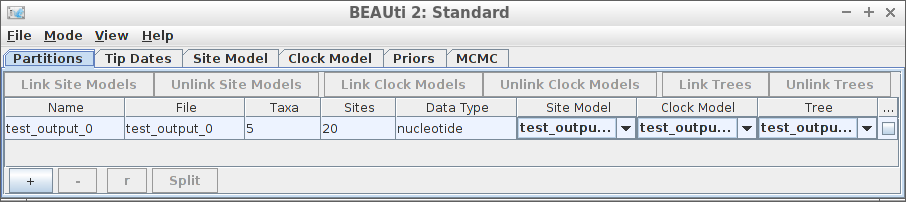Example #1: all default

``````if (is_beast2_installed()) {
inference_model <- create_inference_model(
mcmc = mcmc
)
beast2_options <- create_beast2_options()
posterior <- bbt_run_from_model(
fasta_filename = fasta_filename,
inference_model = inference_model,
beast2_options = beast2_options
)
bbt_delete_temp_files(
inference_model = inference_model,
beast2_options = beast2_options
)
}``````

All other parameters are set to their defaults, as in BEAUti.

``plot_densitree(posterior\$anthus_aco_sub_trees, width = 2)``## Example #2: using an MRCA prior to specify a crown age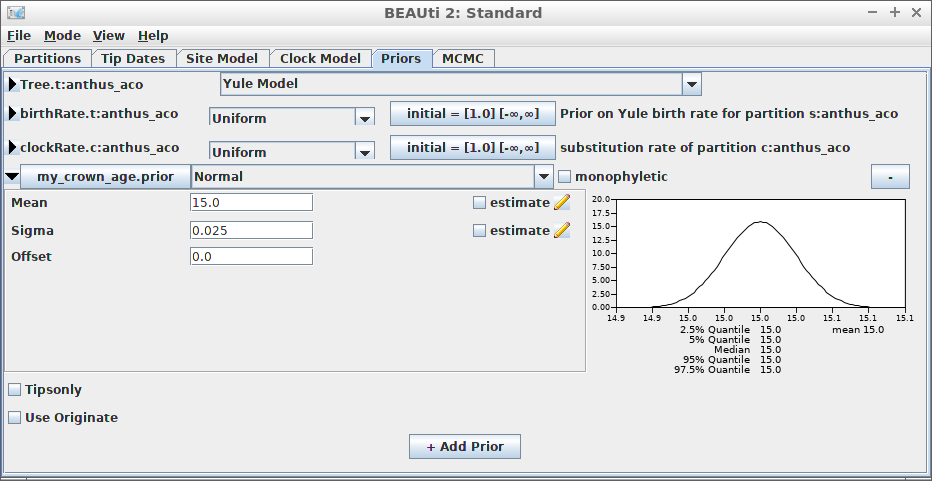Example #2: using an MRCA prior to specify a crown age

An alternative is to date the node of the most recent common ancestor of all taxa.

Create the MCMC:

``mcmc <- create_test_mcmc(chain_length = 10000)``
``````if (is_beast2_installed()) {
inference_model <- create_inference_model(
mcmc = mcmc,
mrca_prior = create_mrca_prior(
taxa_names = sample(get_taxa_names(fasta_filename), size = 3),
alignment_id = get_alignment_id(fasta_filename),
is_monophyletic = TRUE,
mrca_distr = create_normal_distr(
mean = 15.0,
sigma = 0.025
)
)
)
beast2_options <- create_beast2_options()
posterior <- bbt_run_from_model(
fasta_filename = fasta_filename,
inference_model = inference_model,
beast2_options = beast2_options
)
bbt_delete_temp_files(
inference_model = inference_model,
beast2_options = beast2_options
)
}``````

Here we use an MRCA prior with fixed (non-estimated) values of the mean and standard deviation for the common ancestor node’s time.

``plot_densitree(posterior\$anthus_aco_sub_trees, width = 2)``## Example #3: JC69 site model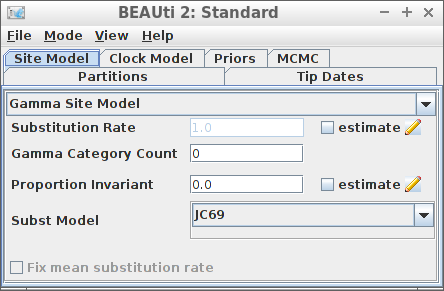Example #3: JC69 site model

``````if (is_beast2_installed()) {
inference_model <- create_inference_model(
site_model = create_jc69_site_model(),
mcmc = mcmc
)
beast2_options <- create_beast2_options()
posterior <- bbt_run_from_model(
fasta_filename = fasta_filename,
inference_model = inference_model,
beast2_options = beast2_options
)
bbt_delete_temp_files(
inference_model = inference_model,
beast2_options = beast2_options
)
}``````
``plot_densitree(posterior\$anthus_aco_sub_trees, width = 2)``## Example #4: Relaxed clock log normal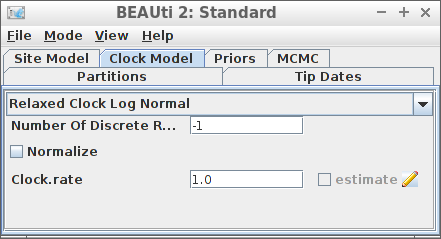Example #4: Relaxed clock log normal

``````if (is_beast2_installed()) {
inference_model <- create_inference_model(
clock_model = create_rln_clock_model(),
mcmc = mcmc
)
beast2_options <- create_beast2_options()
posterior <- bbt_run_from_model(
fasta_filename = fasta_filename,
inference_model = inference_model,
beast2_options = beast2_options
)
bbt_delete_temp_files(
inference_model = inference_model,
beast2_options = beast2_options
)
}``````
``plot_densitree(posterior\$anthus_aco_sub_trees, width = 2)``## Example #5: Birth-Death tree prior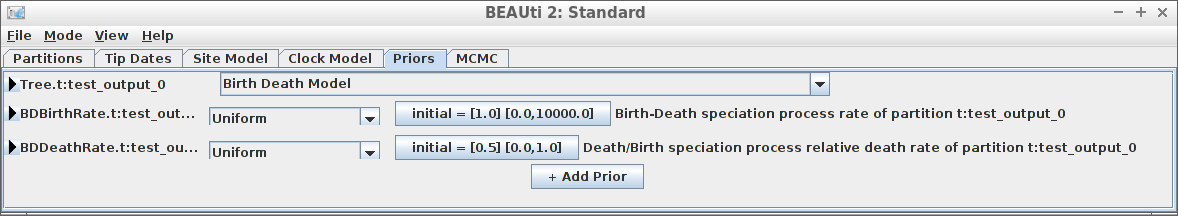Example #5: Birth-Death tree prior

``````if (is_beast2_installed()) {
inference_model <- create_inference_model(
tree_prior = create_bd_tree_prior(),
mcmc = mcmc
)
beast2_options <- create_beast2_options()
posterior <- bbt_run_from_model(
fasta_filename = fasta_filename,
inference_model = inference_model,
beast2_options = beast2_options
)
bbt_delete_temp_files(
inference_model = inference_model,
beast2_options = beast2_options
)
}``````
``plot_densitree(posterior\$anthus_aco_sub_trees, width = 2)``## Example #6: Yule tree prior with a normally distributed birth rate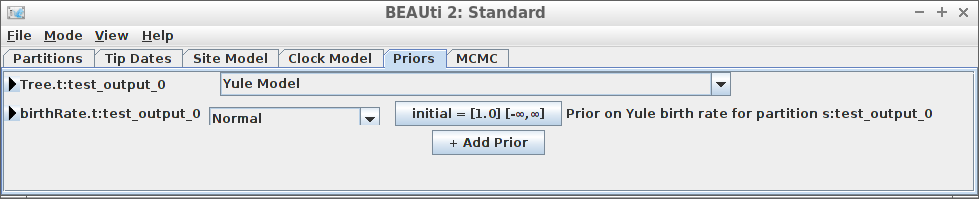Example #6: Yule tree prior with a normally distributed birth rate

``````if (is_beast2_installed()) {
inference_model <- create_inference_model(
tree_prior = create_yule_tree_prior(
birth_rate_distr = create_normal_distr(
mean = 1.0,
sigma = 0.1
)
),
mcmc = mcmc
)
beast2_options <- create_beast2_options()
posterior <- bbt_run_from_model(
fasta_filename = fasta_filename,
inference_model = inference_model,
beast2_options = beast2_options
)
bbt_delete_temp_files(
inference_model = inference_model,
beast2_options = beast2_options
)
}``````
``plot_densitree(posterior\$anthus_aco_sub_trees, width = 2)``Thanks to Yacine Ben Chehida for this use case

## Example #7: HKY site model with a non-zero proportion of invariants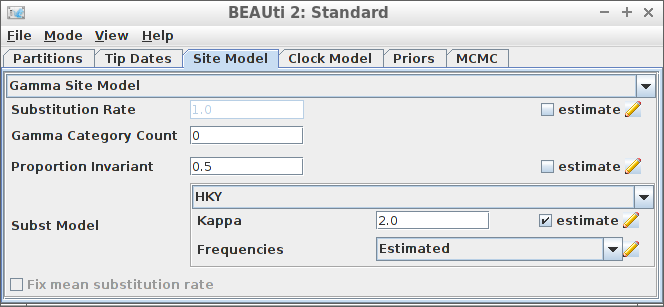Example #7: HKY site model with a non-zero proportion of invariants

``````if (is_beast2_installed()) {
inference_model <- create_inference_model(
site_model = create_hky_site_model(
gamma_site_model = create_gamma_site_model(prop_invariant = 0.5)
),
mcmc = mcmc
)
beast2_options <- create_beast2_options()
posterior <- bbt_run_from_model(
fasta_filename = fasta_filename,
inference_model = inference_model,
beast2_options = beast2_options
)
bbt_delete_temp_files(
inference_model = inference_model,
beast2_options = beast2_options
)
}``````
``plot_densitree(posterior\$anthus_aco_sub_trees, width = 2)``Thanks to Yacine Ben Chehida for this use case

## Example #8: Strict clock with a known clock rate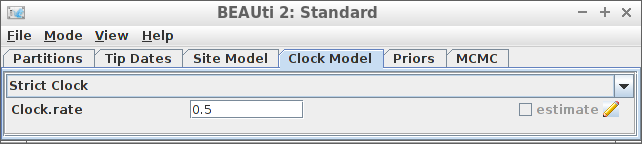Example #8: Strict clock with a known clock rate

``````if (is_beast2_installed()) {
inference_model <- create_inference_model(
clock_model = create_strict_clock_model(
clock_rate_param = 0.5
),
mcmc = mcmc
)
beast2_options <- create_beast2_options()
posterior <- bbt_run_from_model(
fasta_filename = fasta_filename,
inference_model = inference_model,
beast2_options = beast2_options
)
bbt_delete_temp_files(
inference_model = inference_model,
beast2_options = beast2_options
)
}``````
``plot_densitree(posterior\$anthus_aco_sub_trees, width = 2)``Thanks to Paul van Els and Yacine Ben Chehida for this use case.